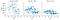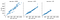# Introduction to Supervised algorithms

Today we will talk about the best known of supervised algorithms: Linear Regression, a classic in machine learning explanations. In addition to understanding the operation of this mathematical algorithm, we will see important concepts to keep in mind, such as generalization and regularization, the overfitting problem and some important metrics and parameters to evaluate and refine the ML model.

But what is a supervised algorithm in ML? The keyword supervised comes from the idea of ​​having a previously labeled and classified data set. Let’s say that the algorithm receives certain characteristics (features) of the data, and also the value of the response (labels), then you must combine them in order to make predictions. There are 2 types of supervised learning:

• Regression, the labels are a numerical value, and with the variables of features, digits will be obtained as the resulting data.

# Linear Regression

Linear regression models fit linear functions to data, like the y=mx+b equations we learned in algebra. Expresses the output value (F(x) or y — target) in terms of a sum of weighted input variables (X).

So for example, if F(x) is vehicle price, and x1 is age model and x2 is HP engine, then we can predict the vehicle price.

F(x) = w1⋅x1 + w2⋅x2 + b

b is a bias constant or model intercept.

The slope indicates the steepness of a line and the intercept indicates the location where it intersects an axis. The slope and the intercept define the linear relationship between two variables.

Ordinary least squares

The method of least squares is used to find the best-fitting line for the observed data. The estimated least squares regression equation has the minimum sum of squared errors, or deviations, between the fitted line and the observations.

This method can work well for data with many features, although it does not have parameters to control the complexity of the model and make it generalize better.

Generalization

It is the ability of a ML model to make accurate predictions on new data not previously seen, that is, on data with which it was not trained. Overfitting problem It happens when the model is tuned very specifically to the training set. Models that are too complex for the training dataset are told that they are overfitted so they will not generalize well, and have high variance ( the difference in fitting between Training and Testing) On the other hand, simple models that do not respond well to the training data are underfitting and will not generalize well either.

Understanding, detecting and avoiding overfitting is the most important aspect of a supervised ML algorithm that we must master.

# Let’s analyze our data

Ok, enough explanation of the linear regression model. In our well-known bike riders dataset, we have built a new dataset that groups the number of daily trips, the total time it took to make those trips, grouped by type of day (obtained in the k-means example), plus the average temperature of the day.

The trips are grouped according to the time of the trip: laboral day rush hour, laboral non-rush hour and weekends / holidays.

The goal of our ML model will be to predict the variable that represents the amount of total daily time spent in bike trips ( hs_day, target-y ), according to a certain day type (LPH, LNPH, WE).

First, we import the necessary libraries and open the data file in our jupyter notebook:

`import matplotlib.pyplot as plt import pandas as pd import numpy as np import seaborn as sns from sklearn.model_selection import train_test_split from sklearn.linear_model import LinearRegression from sklearn.metrics import mean_squared_error, r2_score from sklearn import preprocessing %matplotlib notebookbr_data = pd.read_csv('./br2018_resume.csv')`

Check for NaNs in tavg column (returned 13 rows) and rename duration_count to give a more correct name to that feature (I include this type of code in pandas to have more know how about the library)

`# check for nan values in column br_data['tavg'].isna().sum() # put before row value in NaN values br_data['tavg'].bfill(axis=0, inplace=True) # rename duration_count = bike rides per day br_data.rename(columns={'duration_count': 'bike_rides_day'}, inplace=True)`

Let’s visualize the data we have, grouped by type of day, in order to examine correlations

`# hours bike ride per day VS avg.temp. g = sns.FacetGrid(br_data, col="type_day") g.map(sns.scatterplot, "tavg", "hs_day", alpha=.7) g.add_legend()`hours bike rides a day vs avg.temp (degrees)
`# bike rides per day (count) VS avg.temp. g = sns.FacetGrid(br_data, col="type_day") g.map(sns.scatterplot, "bike_rides_day", "hs_day", alpha=.7) g.add_legend()`hours bike rides a day vs bike rides travels a day (avg. travel about 20–30 minutes)

We will focus on the results of the LPH day type. In graph A, we visualize that the linear correlation is stronger than in the other two groups and maintains a downward trend or negative correlation, so the higher the temperature, the fewer hours of bike rides. In graph B, we visualize an almost perfect linear correlation with a clear upward trend or positive correlation. In this case we must understand that we are correlating 2 characteristics of the data that arise from the same data: bicycle trips, which is why it is logical that the greater the number of trips, the greater the amount of time. Later we will incorporate this feature (bike_rides_day) to the model to improve its precision.

Let’s see now how seaborn lmplot graph allows us to visualize the regression lines of the data from graph A for all types of days.

# Build our linear regression model

Now we will use sklearn to create a linear regression model and apply it initially on a single feature, then we will evaluate the precision of our model.

`#Build 3 df , one per day type df_WE = br_data[br_data['type_day']=='WEND'] df_LPH = br_data[br_data['type_day']=='LPH'] df_LNPH = br_data[br_data['type_day']=='LNPH']  # X only with 1 feature X = df_LPH[['tavg']] y = df_LPH['hs_day'] X_train, X_test, y_train, y_test = train_test_split(X, y)  # create linear regression model, fit and predict  regressor = LinearRegression() regressor.fit(X_train, y_train) y_pred = regressor.predict(X_test)`

Let’s stop here, sklearn provides us with the train_test_split method to generate two datasets, one containing data to train the model and the other to test it, by default 75% for training and the remaining 25% for testing. Later we create the linear regression model and train it (fit ) finally we predict the values ​​on the test set. In this case, the model was trained with 183 instances and then tested with 61.

As precision metric we will use the r2 score, known as the coefficient of determination. In few words, the coefficient determines the quality of the model to replicate the results, and the proportion of variation of those results that can be explained by the model.

`model_r2_score = regressor.score(X_test, y_test) print(f'R-squared: {model_r2_score}' )`

R-squared: 0.19552131529170358, in this case, our value of R2 is a little low, knowing that 1 is the optimal one. The obvious solution is to add more features to our model …

`# X with 2 features X2 = df_LPH[['bike_rides_day','tavg']] y2 = df_LPH['hs_day'] X2_train, X2_test, y2_train, y2_test = train_test_split(X2, y2)  # create linear regression for 2 features in X, fit and predict  regressor2 = LinearRegression() regressor2.fit(X2_train, y2_train) y2_pred = regressor2.predict(X2_test) model_r2_score = regressor2.score(X2_test, y2_test) print(f'R-squared: {model_r2_score}' )`

R-squared: 0.9597724505319959, now we have obtained an impressive value that allows us to be optimistic about our model.

Since we have carried out our training and test executed on a single data division, each time we execute a new train_test_split we will obtain different precision values. To correct this, we have the cross-validation method, which is a technique that guarantees the independence of the partition between training and test data. This repeats and calculates the evaluation metric on different partitions (cv parameter)

`cv_scores = cross_val_score(regressor2, X2, y2, cv=6) print(f'CV scores list: {cv_scores}') print(f'Mean R-squared with 6 CV scores: {np.mean(cv_scores)}' )`

CV scores list: [0.40073867 0.95355093 0.92916654 0.97397186 0.83496845 0.83116272]

Mean R-squared with 6 CV scores: 0.8205931950492525, is still a promising value, so we will end our evaluation of the model.

Remember that with linear regression there are 2 important values ​​that the model calculates to obtain the output function: slope and intercept. We can visualize such values ​​of our model as follows:

`coef = regressor2.coef_ intercept = regressor2.intercept_ print(f'Intercept: {intercept} - Slope: {coef}')`

Intercept: -149.06483526276838 — Slope: [0.38677462 5.96006047]

Another important question has to do with something that we named at the beginning, and that is that ordinary linear regression does not have any parameter to control the complexity of the model and thus achieve a greater generalization of it. One solution to this is to apply Ridge Regression

L2 Ridge regression

Is a Regularization method to reduce overfitting. Uses the same least squares criterion but adds a penalty for large variations in the values ​​of w.

Regularization avoids overfitting and improves generalization, in this case it is the L2 penalty added to the model. What we do in practice, is to introduce a Bias that we call Lambda, and the Penalty Function is: lambda*slope² ( L2 ).

The value of the regularization is controlled by the alpha parameter (default 10), the higher it implies more regularization

`# Ridge regression, add regularization with alpha value (default 10) from sklearn.linear_model import Ridge ridge_regressor = Ridge(alpha=20.0).fit(X2_train, y2_train) print(f'R-squared: {ridge_regressor.score(X2_test, y2_test)}' )`

R-squared: 0.9597612729666329

In this case we obtain an almost identical value to ordinary linear regression, but in some very overfitted models it can help a lot.

There is another type of regression with penalty, called Lasso Regression, which uses an L1 penalty, which minimizes the sum of the absolute values ​​of the coefficients, achieving that a subset of them is forced to be 0. The level of regularization is managed with the alpha parameter (default = 0)

# Visualization of model results

Now we will build a graph that allows us to visualize the prediction results of the model against the actual results of the test set.

So we will build a new dataframe that will contain the values ​​of y (hs_day) predicted by the system and the real data from the test set, and thus be able to compare the prediction differences.

`# join in a df the X,y test values df_test = X2_test.join(y2_test) df_test['data_type'] = 'Test' df_test.reset_index(drop=True, inplace=True) # join in a df the X test values with y predictions df_pred = X2_test.join(y2_pred_series) df_pred['data_type'] = 'Pred' df_pred.reset_index(drop=True, inplace=True) `
`# concatenate both df (df_test + df_pred) df_test_pred = pd.concat([df_test, df_pred]) # Build 3d graph with df_test_pred data import plotly.express as px fig = px.scatter_3d(df_test_pred, x="bike_rides_day", y="tavg",                                     z="hs_day", color='data_type', symbol='data_type', )fig.update_layout(margin=dict(l=0, r=20, b=20, t=0)) fig.show()`

The blue circles correspond to the actual data from the test set, and the red diamonds to the predicted data. In the interactive 3d graph you could position yourself with the cursor over any point and see the values ​​obtained.

For example for: bike_rides_day = 5034 and tavg = 21.2, y (hs_day) real test value (blue) = 1975.37. While for y (hs_day) predicted (red) = 1924.31

As always the github link with the complete linear regression jupyter notebook is attached so that you can verify the code for yourself.

Ok folks, now is time to generate your own Linear Regression model project with Scikit-Learn and advance your data science career.

Also follow me on my data science blog EmpowereDataScience

Your comments are appreciated

I am a senior python programmer and a professor, passionate about data science and everything related to artificial intelligence, and the space race

## More from Jorge Ercoli

I am a senior python programmer and a professor, passionate about data science and everything related to artificial intelligence, and the space race